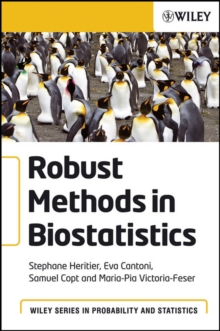Supporting your high street Find out how »
• My Account# Robust Methods in Biostatistics Hardback

## Part of the Wiley Series in Probability and Statistics series

#### Description

Robust statistics is an extension of classical statistics that specifically takes into account the concept that the underlying models used to describe data are only approximate.

Its basic philosophy is to produce statistical procedures which are stable when the data do not exactly match the postulated models as it is the case for example with outliers. Robust Methods in Biostatistics proposes robust alternatives to common methods used in statistics in general and in biostatistics in particular and illustrates their use on many biomedical datasets.

The methods introduced include robust estimation, testing, model selection, model check and diagnostics.

They are developed for the following general classes of models: Linear regression Generalized linear models Linear mixed models Marginal longitudinal data models Cox survival analysis model The methods are introduced both at a theoretical and applied level within the framework of each general class of models, with a particular emphasis put on practical data analysis.

This book is of particular use for research students,applied statisticians and practitioners in the health field interested in more stable statistical techniques. An accompanying website provides R code for computing all of the methods described, as well as for analyzing all the datasets used in the book.

#### Information

• Format: Hardback
• Pages: 294 pages
• Publisher: John Wiley & Sons Inc
• Publication Date:
• Category: Probability & statistics
• ISBN: 9780470027264

£82.75

£69.49£60.00

£51.39

£102.00

£91.15

£106.00

£94.65